Courses
Courses for Kids
Free study material
Offline Centres
More

# NCERT Solutions for Class 12 Chemistry Chapter 10 - In HindiLast updated date: 05th Dec 2023
Total views: 426.6k
Views today: 5.26k

## NCERT Solutions for Class 12 Chemistry Chapter 10 Haloalkanes and Haloarenes in Hindi Mediem

Download the Class 12 Chemistry NCERT Solutions in Hindi medium and English medium as well offered by the leading e-learning platform Vedantu. If you are a student of Class 12, you have reached the right platform. The NCERT Solutions for Class 12 Chemistry in Hindi provided by us are designed in a simple, straightforward language, which are easy to memorise. You will also be able to download the PDF file for NCERT Solutions for Class 12 Chemistry  in Hindi from our website at absolutely free of cost.

NCERT, which stands for The National Council of Educational Research and Training, is responsible for designing and publishing textbooks for all the classes and subjects. NCERT textbooks covered all the topics and are applicable to the Central Board of Secondary Education (CBSE) and various state boards.

We, at Vedantu, offer free NCERT Solutions in English medium and Hindi medium for all the classes as well. Created by subject matter experts, these NCERT Solutions in Hindi are very helpful to the students of all classes.

## Access NCERT Solutions for Class 12 Chemistry Chapter 10 – हैलोऐल्केन तथा हैलोऐरीन

1. निम्नलिखित हैलाइडों के नाम आई०यू०पी०ए०सी० (IUPAC) पद्धति से लिखिए तथा उनका वर्गीकरण, ऐल्किल, ऐलिलिक, बेन्जिलिक (प्राथमिक, द्वितीयक एवं तृतीयक), वाइनिल अंथवा ऐरिल हैलाइड के रूप में कीजिए –

1. ${\mathbf{\left( {C{H_3}} \right)_2}CHCH\left( {Cl} \right)C{H_3}}$

उत्तर: $2 -$क्लोरो$- 3 -$मेथिलब्यूटेन, $2^\circ$ ऐल्किल हैलाइड

2. $\mathbf{C{H_3}C{H_2}CH\left( {C{H_3}} \right)CH\left( {{C_2}{H_5}} \right)Cl}$

उत्तर: $3 -$क्लोरो$- 4$ मेथिलहेक्सेन, $2^\circ$ ऐल्किल हैलाइड

3. $\mathbf{C{H_3}C{H_2}C{\left( {C{H_3}} \right)_2}C{H_2}}I$

उत्तर: $1 -$आयोडो$- 2,{\text{ }}2 -$डाइमेथिलब्यूटेन,$1^\circ$ ऐल्किल हैलाईड

4. $\mathbf{{\left( {C{H_3}} \right)_3}CC{H_2}CH\left( {Br} \right){C_6}{H_5}}$

उत्तर:$1 -$ ब्रोमो$- 3,{\text{ }}3 -$डाइमेथिल$- 1 -$ फेनिलब्यूटेन, $2^\circ$बेन्जिलिक हैलाइड

5. $\mathbf{{\text{C}}{{\text{H}}_{\text{3}}}{\text{CH}}\left( {{\text{C}}{{\text{H}}_{\text{3}}}} \right){\text{CH}}\left( {{\text{Br}}} \right){\text{C}}{{\text{H}}_{\text{3}}}}$

उत्तर: $2 -$ ब्रोमो$- 3 -$मेथिलब्यूटेन, $2^\circ$ऐल्किल हैलाइड

6.$\mathbf{{\text{C}}{{\text{H}}_{\text{3}}}{\text{C}}{\left( {{{\text{C}}_{\text{2}}}{{\text{H}}_{\text{5}}}} \right)_{\text{2}}}{\text{C}}{{\text{H}}_{\text{2}}}{\text{Br}}}$

उत्तर:$1 -$ब्रोमो$- 2 -$एथिल$- 2 -$मेथिलब्यूटेन, $1^\circ$ऐल्किल हैलाइड

7.$\mathbf{C{H_3}C\left( {Cl} \right)\left( {{C_2}{H_5}} \right)C{H_2}C{H_3}}$

उत्तर:$3 -$क्लोरो$- 3 -$मेथिलपेन्टेन, $3^\circ$ऐल्किल हैलाइड

8.$\mathbf{C{H_3}CH{\text{ }} = {\text{ }}C\left( {Cl} \right)C{H_2}CH{\left( {C{H_3}} \right)_2}}$

उत्तर:$3 -$क्लोरो$- 5 -$मेथिलहेक्स$- 2 -$ईन,वाइनिलिक हैलाइड

9.$\mathbf{C{H_3}CH{\text{ }} = {\text{ }}CHC\left( {Br} \right){\left( {C{H_3}} \right)_2}}$

उत्तर:$4 -$ब्रोमो$- 4 -$मेथिलपेन्ट$- 2 -$ईन, ऐलीलिक हैलाइड

10.$\mathbf{p - Cl{C_6}{H_4}C{H_2}CH{\left( {C{H_3}} \right)_2}}$

उत्तर: $1 -$क्लोरो$- 4 - (2 -$मेथिलप्रोपिल)- बेंजीन, ऐरिल हैलाइड

11.$\mathbf{m - ClC{H_2}{C_6}{H_4}C{H_2}C{\left( {C{H_3}} \right)_3}}$

उत्तर:$1 -$क्लोरोमेथिल$- 3 - (2,{\text{ }}2 -$डाइमेथिलप्रोपिल) बेंजीन, $1^\circ$बेंजाइलिक हैलाइड

12.$\mathbf{o - Br - {C_6}{H_4}CH\left( {C{H_3}} \right)C{H_2}C{H_3}}$

उत्तर:$1 -$ब्रोमो$- 2 - (1 -$मेथिलप्रोपिल) बेंजीन, ऐरिल हैलाइड

2. निम्नलिखित यौगिकों के IUPAC नाम दीजिए –

$\mathbf{1.C{H_3}CH\left( {Cl} \right)CH\left( {Br} \right)C{H_3}}$

उत्तर:$2 -$ब्रोमो$- 3 -$क्लोरोब्यूटेन

$\mathbf{2.CH{F_2}CBrClF}$

उत्तर:$1 -$ब्रोमो$- 1 -$क्लोरो$- 1,{\text{ }}2,{\text{ }}2 -$ट्राइफ्लोरोएथेन

$\mathbf{3.ClC{H_2}C{\text{ }} \equiv {\text{ }}CC{H_2}Br}$

उत्तर: $1 -$ब्रोमो$- 4 -$क्लोरोब्यूट$- 2 -$आइन

$\mathbf{4.\left( {{\mathbf{CC}}{{\mathbf{l}}_{\mathbf{3}}}} \right){\mathbf{3CCl}}}$

उत्तर: $2 -$(ट्राइक्लोरोमेथिल) $- 1,1,1,{\text{ }}2,{\text{ }}3,{\text{ }}3,{\text{ }}3, -$हैप्टाक्लोरोप्रोपेन

$\mathbf{5.C{H_3}C{\left( {p - {\text{ }}Cl{C_6}{H_4}} \right)_2}CH\left( {Br} \right)C{H_3}}$

उत्तर: $2 -$ब्रोमो$- 3,3 -$बिस ($4 -$क्लोरोफेनिल)ब्यूटेन

$\mathbf{6.\left( {{\mathbf{C}}{{\mathbf{H}}_{\mathbf{3}}}} \right){\mathbf{3CCH}}{\text{ }} = {\text{ }}{\mathbf{ClC}}_{\mathbf{6}}^{}{{\mathbf{H}}_{\mathbf{4}}}{\text{ }}{\mathbf{I}} - {\mathbf{P}}}$

उत्तर: $1 -$क्लोरो$- 1 -$($4 -$आयोडोफेनिल)$- 3,{\text{ }}3 -$डाइमेथिलब्यूट$- {\text{ }}1 -$ईन

3. निम्नलिखित कार्बनिक हैलोजेन यौगिकों की संरचना दीजिए –

(i) $2 -$क्लोरो$- 3 -$मेथिलपेन्टेन

उत्तर: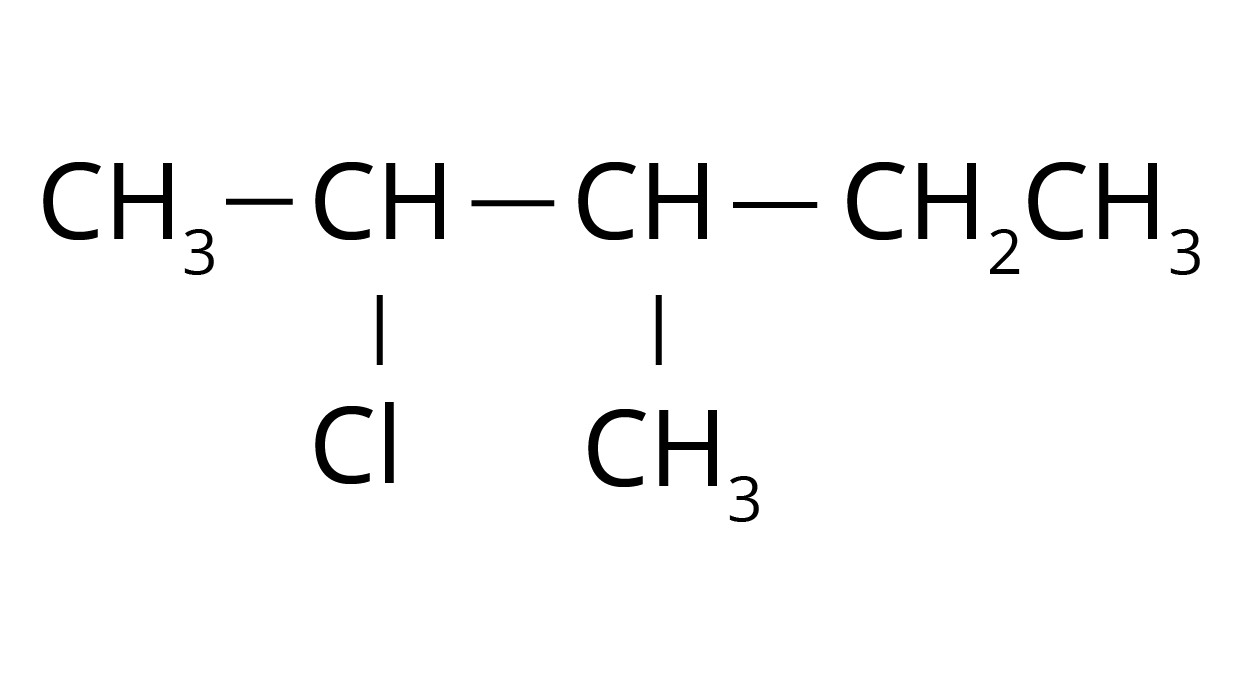(ii) p-ब्रोमोक्लोरो बेन्जीन

उत्तर: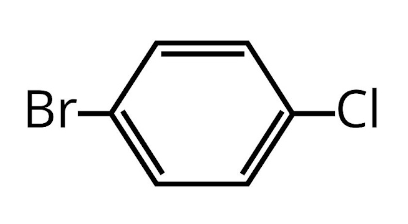(iii) $1 -$क्लोरो$- 4 -$एथिलसाइक्लोहेक्सेन

उत्तर: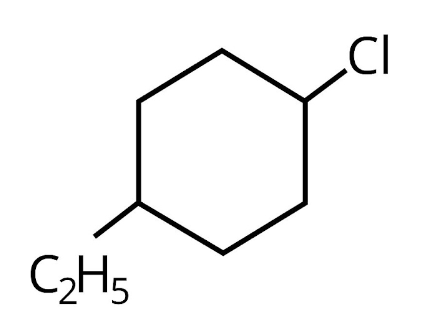(iv) $2 -$($2 -$क्लोरोफेनिल)$- 1 -$आयोडोऑक्टेन

उत्तर: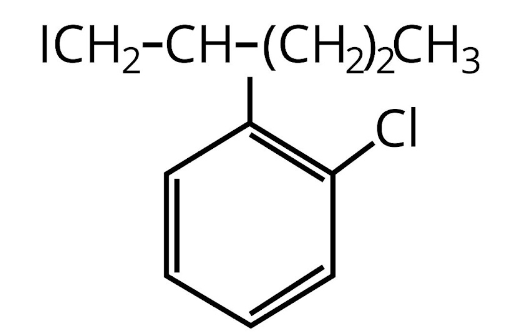(v) $2 -$ब्रोमोब्यूटेन

उत्तर: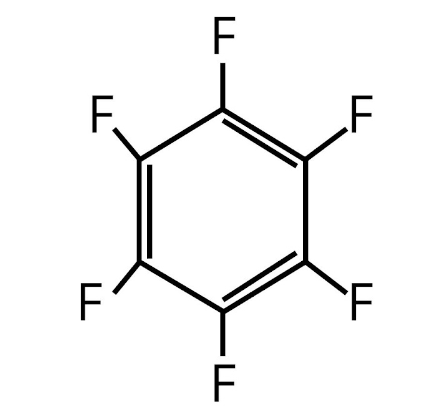(vi) $4 -$तृतीयक-ब्यूटिल$- 3 -$आयोडोहेप्टेन

उत्तर: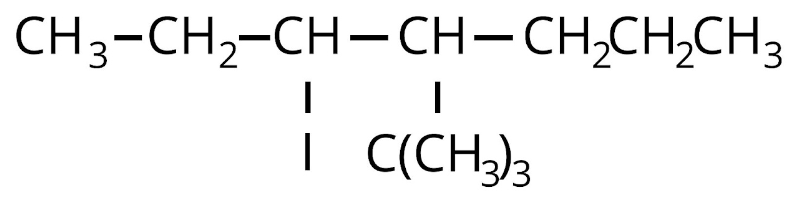(vii) $1 -$ब्रोमो$- 4 -$द्वितीयक-ब्यूटिल$- 2 -$मेथिल बेन्जीन

उत्तर: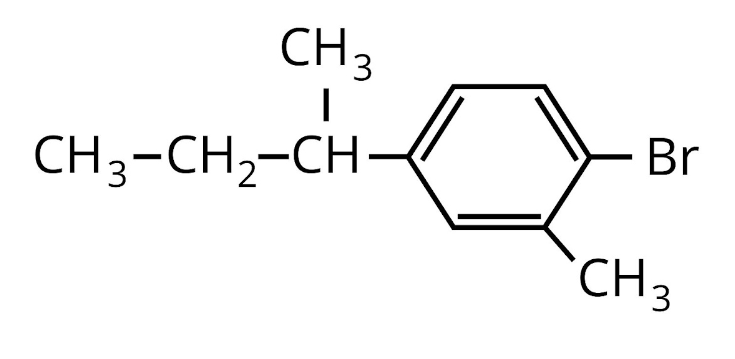(viii) $1,4 -$डाइब्रोमोब्यूट$- 2 -$ईन।

उत्तर: ${\text{BrC}}{{\text{H}}_2}{\text{CH}} = {\text{CHC}}{{\text{H}}_2}{\text{Br}}$

4. निम्नलिखित में से किसका द्विध्रुव आघूर्ण सर्वाधिक होगा?

1. $\mathbf{C{H_2}C{l_2}}$

उत्तर: $C{H_2}C{l_2}$ का द्विध्रुव आघूर्ण सर्वाधिक है। इसका कारण यह है कि इसमें दोनों ${\text{C - Cl}}$आबन्ध आघूर्गों का परिणामी तथा दोनों

C-H आबन्ध आघूर्गों के परिणामी एक ही दिशा में कार्य करते हैं।

2. $\mathbf{CHC{l_3}}$

उत्तर:$CHC{l_3}$ में दोनों ${\text{C - Cl}}$आबन्ध आघूर्गों का परिणामी तीसरे ${\text{C - Cl}}$ आबन्ध आघूर्ण के विपरीत दिशा में कार्य करता है।

3. $\mathbf{CC{l_4}}$

उत्तर:$CC{l_4}$ की आकृति सममित होने के कारण इसका द्विध्रुव आघूर्ण शून्य है।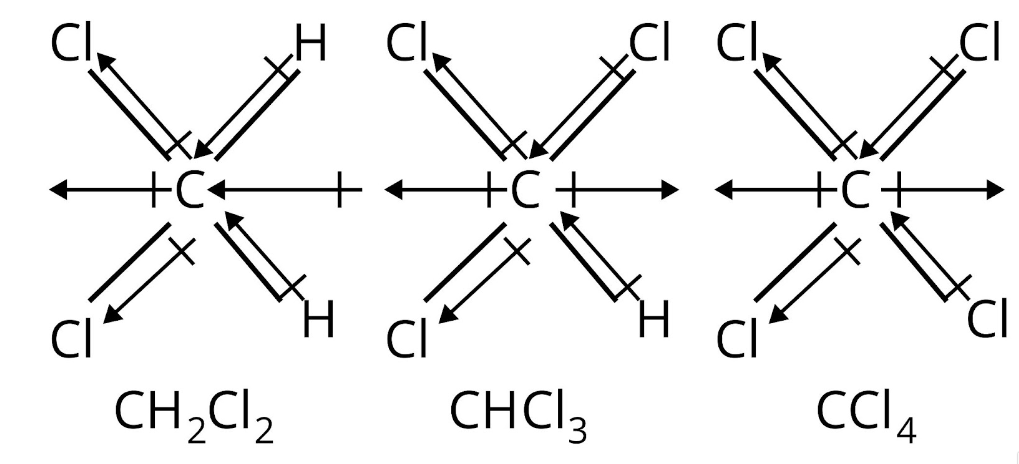5. एक हाइड्रोकार्बन ${C_5}{H_{10}}$ अँधेरे में क्लोरीन के साथ अभिक्रिया नहीं करता, परन्तु सूर्य के तीव्र प्रकाश में केवल एक मोनोक्लोरो यौगिक ${C_5}{H_9}Cl$ देता है। हाइड्रोकार्बन की संरचना क्या है?

उत्तर: हाइड्रोकार्बन ${C_5}{H_{10}}$ या तो एक ऐल्कीन है अथवा साइक्लोऐल्केन। चूँकि यह क्लोरीन से अन्धेरे में क्रिया नहीं करता है, अतएव यह ऐल्कीन नहीं हो सकता। इस प्रकार इसे एक साइक्लोऐल्केन होना चाहिए। साइक्लोऐल्केन सूर्य के प्रकाश की उपस्थिति में $C{l_2}$ से अभिक्रिया करके मोनोक्लोरो यौगिक, ${C_5}{H_9}Cl$देता है। इसलिए साइक्लोऐल्केन के सभी ${\text{10 H - }}$परमाणु तुल्य होंगे। अत: साइक्लोऐल्केन साइक्लोपेन्टेन (cyclopentane) है। सूर्य के प्रकाश में साइक्लोपेन्टेन निम्न प्रकार से क्लोरीन से क्रिया कर एक मोनोक्लोरो यौगिक ${C_5}{H_9}Cl$ देता है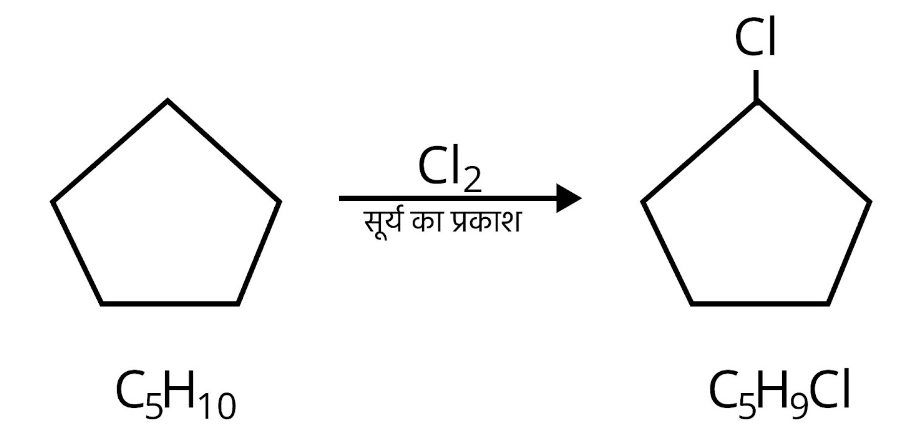इस प्रकार ${C_5}{H_{10}}$ साइक्लोपेन्टेन है।

6. ${C_4}{H_9}Br$ सूत्र वाले यौगिक के सभी समावयवी लिखिए।

उत्तर: ${C_4}{H_9}Br$ एक संतृप्त यौगिक है, क्योंकि इसका पितृ हाइड्रोकार्बन ${C_4}{H_{10}}$ है। इसके समावयवी निम्न हैं –

${\text{C}}{{\text{H}}_3} - {\text{C}}{{\text{H}}_2} - {\text{C}}{{\text{H}}_2} - {\text{C}}{{\text{H}}_2}{\text{Br}}$

$1 -$ब्रोमोब्यूटेन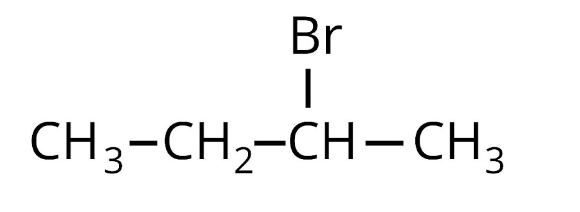$2 -$ब्रोमोब्यूटेन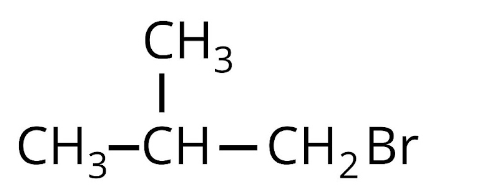$1 -$ब्रोमो$- 2 -$मेथिलप्रोपेन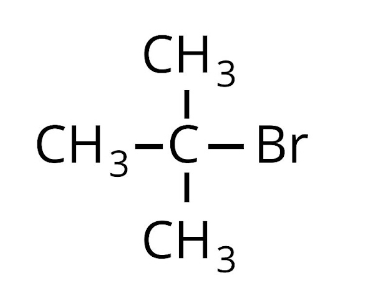$2 -$ब्रोमो$- 2 -$मेथिलप्रोपेन

7. निम्नलिखित से $1 -$आयोडोब्यूटेन प्राप्त करने की समीकरण दीजिए –

(i) $1 -$ब्यूटेनॉल

उत्तर: ${\text{C}}{{\text{H}}_3}{\text{C}}{{\text{H}}_2}{\text{C}}{{\text{H}}_2}{\text{C}}{{\text{H}}_2}{\text{OH}}\mathop \to \limits^{{\text{P}} + {{\text{I}}_2}} {\text{C}}{{\text{H}}_3}{\text{C}}{{\text{H}}_2}{\text{C}}{{\text{H}}_2}{\text{I}}$

$1 -$ब्यूटेनॉल

$1 -$आयोडोब्यूटेन

(ii) $1 -$क्लोरोब्यूटेन

उत्तर: ${\text{C}}{{\text{H}}_3}{\text{C}}{{\text{H}}_2}{\text{C}}{{\text{H}}_2}{\text{C}}{{\text{H}}_2}{\text{Cl}} + {\text{NaI}} \to$ ${\text{C}}{{\text{H}}_3}{\text{C}}{{\text{H}}_2}{\text{C}}{{\text{H}}_2}{\text{C}}{{\text{H}}_2}{\text{I}} + {\text{NaCl}}$                            फिंकेलस्टीन अभिक्रिया

$1 -$क्लोरोब्यूटेन

(iii) ब्यूट$1 -$ईन।

उत्तर: ${\text{C}}{{\text{H}}_2} = {\text{CH}} - {\text{C}}{{\text{H}}_2} - {\text{C}}{{\text{H}}_3} + {{\text{H}}_2}\mathop \to \limits^{{\text{HBr}},{\text{ }}}$${\text{C}}{{\text{H}}_3} - {\text{C}}{{\text{H}}_2} - {\text{C}}{{\text{H}}_2} - {\text{C}}{{\text{H}}_2}{\text{Br}}\mathop \to \limits^{{\text{ }}\Delta } {\text{C}}{{\text{H}}_3} - {\text{C}}{{\text{H}}_2} - {\text{C}}{{\text{H}}_2} - {\text{C}}{{\text{H}}_2}{\text{I}}$

8. उभयदन्ती नाभिकरागी क्या होते हैं? एक उदाहरण की सहायता से समझाइए।

उत्तर: जो नाभिकरागी अभिकर्मक किसी इलेक्ट्रॉन न्यून केन्द्र पर अपने दो भिन्न परमाणुओं के माध्यम से आक्रमण करने में सक्षम होते हैं, उन्हें उभयदन्ती नाभिकरागी कहा जाता है; जैसे- सायनाइड आयन एक उभयदन्ती नाभिकरागी है, क्योंकि यह निम्न दो अनुनाद संरचनाओं का एक संकर है और C तथा N, दोनों परमाणुओं के माध्यम से इलेक्ट्रॉन न्यून केन्द्र पर आक्रमण करने में सक्षम है।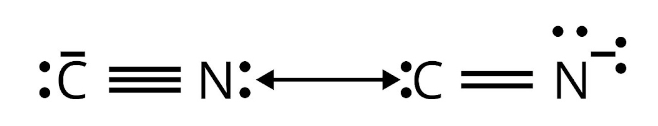9. निम्नलिखित प्रत्येक युग्मों में से कौन-सा यौगिक OH के साथ $S{N_2}$ अभिक्रिया में अधिक तीव्रता से अभिक्रिया करेगा? $C{H_2}Br$ अथवा $C{H_3}I{\text{ }}{\left( {C{H_3}} \right)_3}{\text{ }}CCl$ अथवा CH3Cl

उत्तर: $C{H_3}I$ अधिक तेजी से क्रिया करेगा, क्योंकि ${\text{Br--}}$की अपेक्षा I– आसानी से यौगिक को छोड़ देगा। कम त्रिविम बाधा (steric hindrance) प्रदर्शित करने के कारण $C{H_3}Cl,SN{{\text{ }}_2}$ अभिक्रिया में तेजी से क्रिया करेगा।

10. निम्नलिखित हैलाइडों के एथेनॉल में सोडियम हाइड्रॉक्साइडे द्वारा विहाइड्रोहैलोजेनीकरण के फलस्वरूप बनने वाली सभी ऐल्कीनों की संरचना लिखिए। इसमें से मुख्य ऐल्कीन कौन-सी होगी? ${\mathbf{1}} -$ब्रोमो$- 1 -$मेथिलसाइक्लोहेक्सेन $2 -$क्लोरो$- 2 -$मेथिलब्यूटेन $2,2,3 -$ट्राइमेथिल$- 3 -$ब्रोमोपेन्टेन।

उत्तर:

1. चूँकि Br के दोनों ओर स्थित है-हाइड्रोजन परमाणु समतुल्य हैं, अतएव केवल एक ऐल्कीन प्राप्त होगी।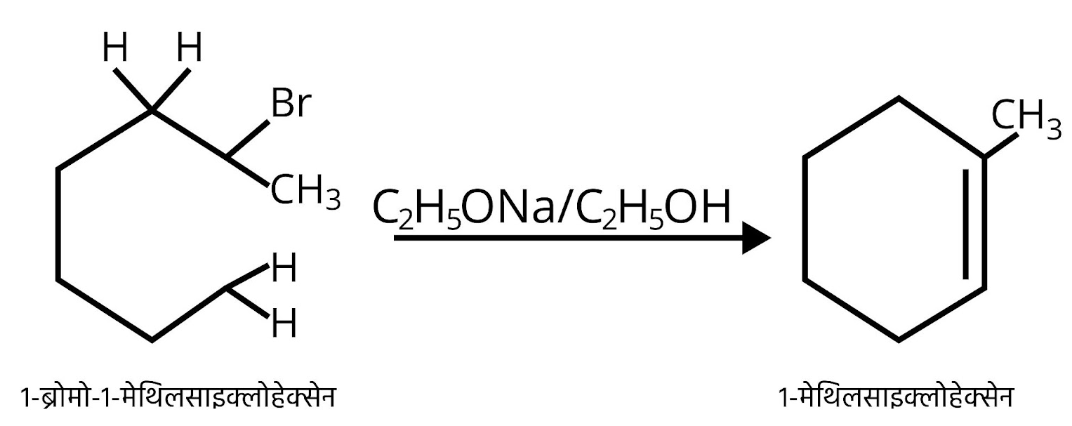1. $2 -$क्लोरो$- 2 -$मेथिलब्यूटेन में समतुल्य β- हाइड्रोजनों के 2 अलग-अलग समुच्चय हैं अत: यह 2 ऐल्कीन देगा।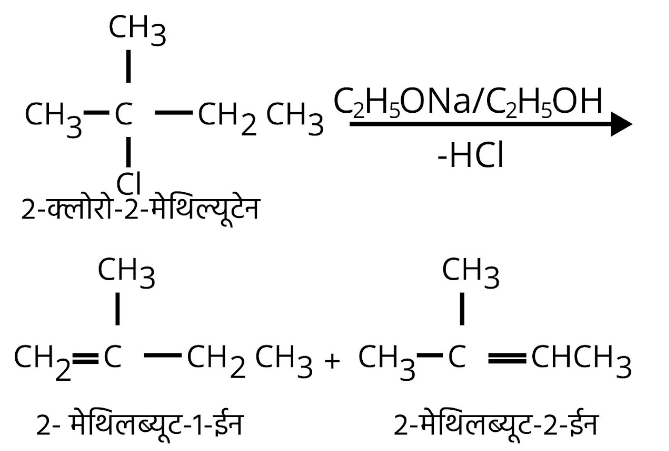चूँकि अधिक प्रतिस्थापित ऐल्कीन अधिक स्थायी होगी अत: $2 -$मेथिलब्यूट$- 2 -$ईन ही मुख्य ऐल्कीन होगी।

1. हैलाइड में दो भिन्न प्रकार के $\beta -$ हाइड्रोजन परमाणु उपस्थित हैं। अतएव विहाइड्रोहैलोजनीकरण अभिक्रिया में यह दो ऐल्कीन $3,4,4 -$ट्राइ-मेथिल पेंट$- 2 -$ईन तथा $2 -$एथिल$- 3$, डाइमेथिलब्यूट$- 1 -$ईन का निर्माण करेगा। पहला ऐल्कीन अधिक स्थिर है, क्योंकि यह अधिक प्रतिस्थापित (more substituted) है (सैटजैफ नियम के अनुसार)। अतएव यह प्रमुख उत्पाद है।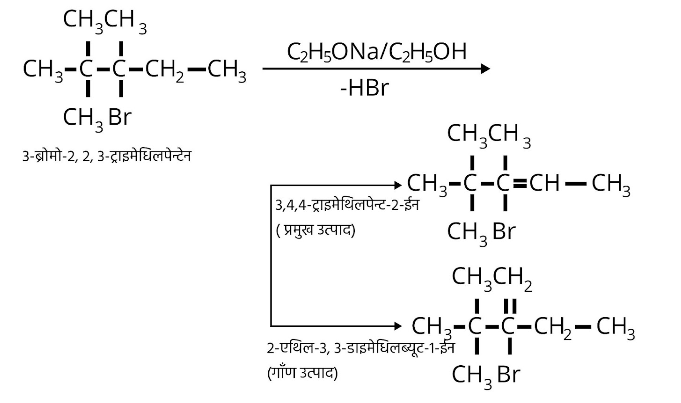11. निम्नलिखित परिवर्तन आप कैसे करेंगे?

(i) एथेनॉल से ब्यूट$- 1 -$आइन

उत्तर:

${\text{C}}{{\text{H}}_3}{\text{C}}{{\text{H}}_2}{\text{OH}}\mathop {\xrightarrow{{SOC{l_2},Pyridine}}}\limits_{ - {\text{S}}{{\text{O}}_2}, - {\text{HCl}}} \mathop {{\text{C}}{{\text{H}}_3}{\text{C}}{{\text{H}}_2} - {\text{Cl}}}\limits_{\text{ }}$

क्लोरोएथेन $(A)$

${\text{HC}} \equiv {\text{CH}} + {\text{NaN}}{{\text{H}}_2}\xrightarrow{{N{H_3}(l),196K}}{\text{HC}} \equiv {{\text{C}}^ - }{\text{N}}{{\text{a}}^ + }$

सोडियम ऐसीटिलाइड $\left( B \right)$

${\text{A}} + {\text{B}} \to {\text{C}}{{\text{H}}_3}{\text{C}}{{\text{H}}_2} - {\text{C}} \equiv {\text{CH}} + {\text{NaCl}}$

ब्यूट$- 1 -$आइन

(ii) एथीन से ब्रोमोएथेन

उत्तर: ${\text{C}}{{\text{H}}_3} - {\text{C}}{{\text{H}}_3} + {\text{B}}{{\text{r}}_2}\xrightarrow{{hv,520 - 670{\text{K}}}}{\text{C}}{{\text{H}}_3}{\text{C}}{{\text{H}}_2} - {\text{Br}} + {\text{HBr}}$

ब्रोमोएथेन

(iii) प्रोपीन से $1 -$नाइट्रोप्रोपीन

उत्तर: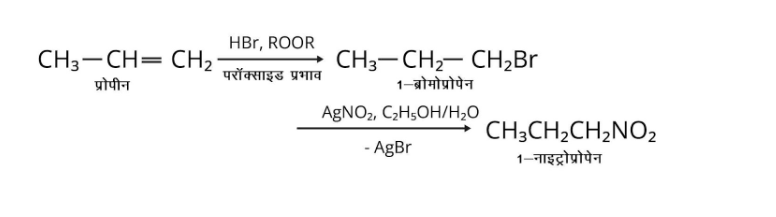(iv) टॉलूईन से बेन्जिल ऐल्कोहॉल

उत्तर:

(v) प्रोपीन से प्रोपाइन

उत्तर: ${\text{C}}{{\text{H}}_3}{\text{CH}} = {\text{C}}{{\text{H}}_2}\mathop {{\text{ - - - - - - > }}}\limits^{{\text{B}}{{\text{r}}_2}/{\text{CC}}{{\text{l}}_4}}$

(vi) एथेनॉल से एथिल फ्लुओराइड

उत्तर: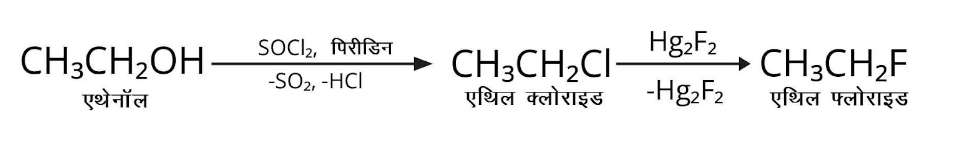(vii) ब्रोमोमेथेन से प्रोपेनोन

उत्तर: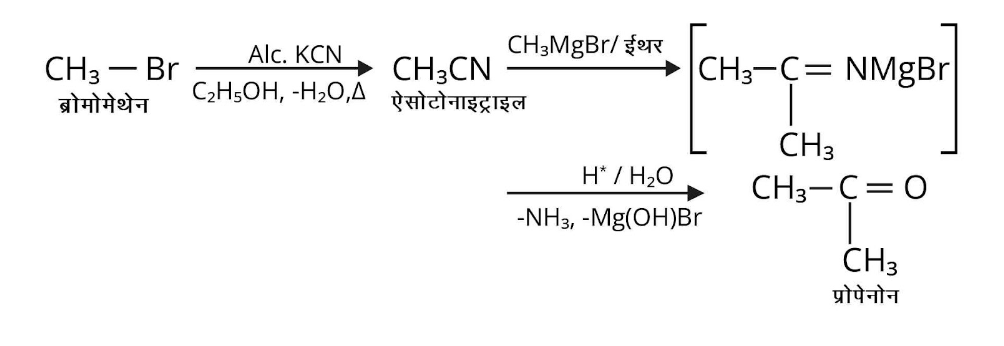(viii) ब्यूट$- 1 -$ईन से ब्यूट$- 2 -$ईन

उत्तर: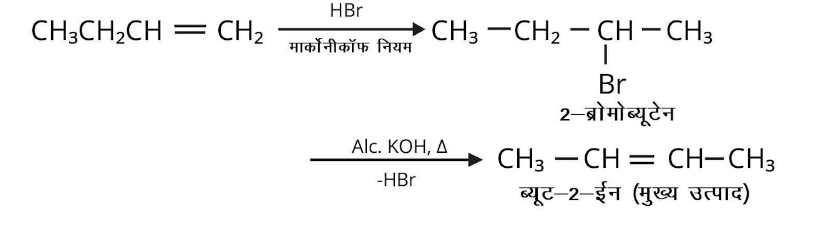(ix) $1 -$क्लोरोब्यूटेन से ${\text{n - }}$ऑक्टेन

उत्तर: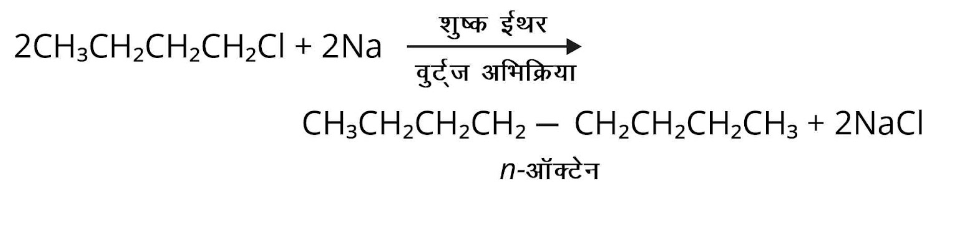(x) बेन्जीन से बाइफेनिल

उत्तर: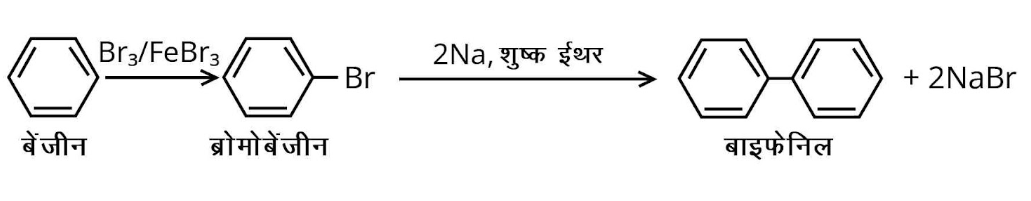12. समझाइए, क्यों – क्लोरोबेन्जीन का द्विध्रुव आघूर्ण साइक्लोहेक्सिले क्लोराइड की तुलना में कम होता है? ऐल्किल हैलाइड ध्रुवीय होते हुए भी जल में अमिश्रणीय हैं? ग्रीन्यार अभिकर्मक का विरचन निर्जलीय अवस्थाओं में करना चाहिए?

उत्तर:

1. उच्च ${\text{s - }}$लक्षण के कारण ${\text{s}}{{\text{p}}^{\text{2}}}{\text{ --}}$संकरित कार्बन ${\text{s}}{{\text{p}}^{\text{3}}}{\text{ --}}$संकरित कार्बन से अधिक ऋणविद्युती होता है। अत: क्लोरोबेंजीन में ${\text{C - Cl}}$ आबन्ध के ${\text{s}}{{\text{p}}^{\text{2}}}{\text{ --}}$संकरित कार्बन में साइक्लोहेक्सिल क्लोराइड के ${\text{s}}{{\text{p}}^{\text{3}}}{\text{ --}}$ ‘संकरित कार्बन की तुलना में ${\text{Cl}}$की इलेक्ट्रॉन मुक्त करने की प्रवृत्ति कम होती है, जिसके परिणामस्वरूप क्लोरोबेंजीन में ${\text{C - Cl}}$ आबन्ध साइक्लोहेक्सिल क्लोराइड में ${\text{C - Cl}}$आबन्ध से कम ध्रुवीय होता है। बेंजीन वलय पर ${\text{Cl}}$ परमाणु के एकाकी युग्म इलेक्ट्रॉनों के विस्थानीकरण (delocalization) के कारण क्लोरोबेंजीन के ${\text{C - Cl}}$ आबन्ध में आंशिक द्विआबन्ध लक्षण आ जाते हैं। दूसरे शब्दों में क्लोरोबेंजीन में ${\text{C - Cl}}$ आबन्ध साइक्लोहेक्सिल क्लोराइड में ${\text{C - Cl}}$ आबन्ध से छोटा होता है। चूँकि द्विध्रुव आघूर्ण आवेश तथा दूरी को गुणनफल होता है, अत: क्लोरोबेंजीन को द्विध्रुव आघूर्ण साइक्लोहेक्सिल क्लोराइड से कम होता है।

2. ऐल्किल हैलाइड ध्रुवीय (polar) होते हैं अत: इनके अणु द्विध्रुव आकर्षण द्वारा परस्पर बँधे रहते हैं। ${{\text{H}}_{\text{2}}}{\text{O}}$के अणु परस्पर हाइड्रोजन आबन्ध द्वारा जुड़े रहते हैं। चूँकि जल तथा ऐल्किल हैलाइड में नये बने आबन्ध बल पूर्व से उपस्थित बलों से दुर्बल होते हैं। अतः ऐल्किल हैलाइड जल में अमिश्रणीय (immiscible) होते हैं। 3. ग्रिगनार्ड (ग्रीन्यार) अभिकर्मक अत्यधिक क्रियाशील होते हैं। ये उपकरण के अन्दर उपस्थित नमी से अभिक्रिया करते हैं। अतः ग्रिगनार्ड अभिकर्मकों को निर्जल परिस्थितियों (anhydrous conditions) में बनाते हैं। $R{\text{ }}--{\text{ }}MgX{\text{ }} + {\text{ }}H{\text{ }}--{\text{ }}OH{\text{ }} \to {\text{ }}RH{\text{ }} + {\text{ }}Mg\left( {OH} \right)X$

13. फ्रेऑन$- 12$, DDT, कार्बन टेट्राक्लोराइड तथा आयोडोफॉर्म के उपयोग दीजिए।

उत्तर: फ्रेऑन$- 12$ के उपयोग (Uses of Freon-12) – यह ऐरोसॉल प्रणोदक, प्रशीतक तथा वायु शीतलन में उपयोग करने के लिए उत्पादित किए जाते हैं।

DDT के उपयोग (Uses of DDT) – DDT का उपयोग कीटनाशी के रूप में किया जाता है, परन्तु जीवों में इसके सतत अन्तर्ग्रहण से उत्पन्न विषैले प्रभावों के कारण इसे प्रतिबन्धित कर दिया गया है। कार्बन टेट्राक्लोराइड के उपयोग (Uses of Carbon Tetrachloride) यह शुष्क धुलाई में विलायक के रूप में प्रयुक्त होता है। यह औषधियों में हुकवर्म तथा कीटनाशक के रूप में प्रयुक्त होता है। यह वसा, तेल, मोम तथा रेजिन के लिए भी उचित विलायक है। आयोडाइड तथा ब्रोमाइड के क्लोरीन जल परीक्षण में भी यह विलायक के रूप में प्रयुक्त किया जाता इससे फ्रेऑन$- 12$ भी प्राप्त होता है। यह पाइरीन नाम से अग्निशामक के रूप में प्रयुक्त होता है। इसके घने वाष्प जलते पदार्थ के ऊपर सुरक्षात्मक परत बनाते हैं और ऑक्सीजन या वायु को जलते पदार्थ के सम्पर्क में आने से रोकते हैं। इसके उपयोग के बाद कक्ष का संवातन (ventilation) करते हैं जिससे बनी हुई फॉस्जीन पूर्णतया दूर हो जाए।

आयोडोफॉर्म के उपयोग (Uses of Iodoform) – इसका उपयोग प्रारम्भ में पूतिरोधी (ऐण्टीसेप्टिक) के रूप में किया जाता था, परन्तु आयोडोफॉर्म का यह पूतिरोधी गुण आयोडोफॉर्म के कारण स्वयं नहीं, बल्कि मुक्त हुई आयोडीन के कारण होता है। इसकी अरुचिकर गन्ध के कारण अब इसके स्थान पर आयोडीनयुक्त अन्य दवाओं का उपयोग किया जाता है।

14. निम्नलिखित प्रत्येक अभिक्रिया में बनने वाले मुख्य कार्बनिक उत्पाद की संरचना लिखिए – (i) $C{H_3}C{H_2}C{H_2}Cl + NaI$ ऐसीटोन  ऊष्मा

उत्तर: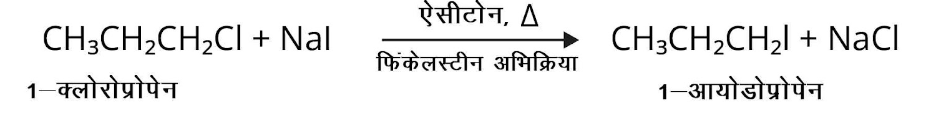(ii) ${\left( {C{H_3}} \right)_3}CBr + KOH$ ऊष्मा  एथेनॉल

उत्तर: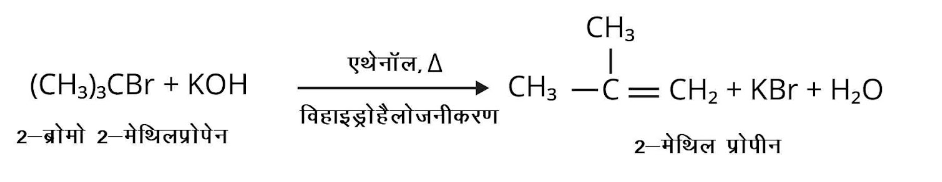(iii) $C{H_3}CH\left( {Br} \right)C{H_2}C{H_3} + NaOH$ जल

उत्तर: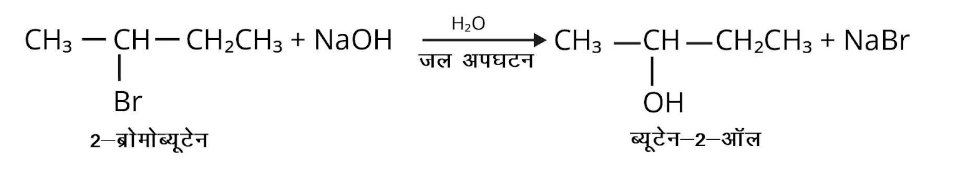(iv) $C{H_3}C{H_2}Br + KCN$ जलीय एथेनॉल

उत्तर: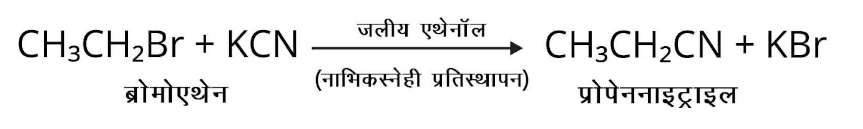(v) ${C_6}{H_5}ONa + {C_2}{H_5}Cl \to$

उत्तर: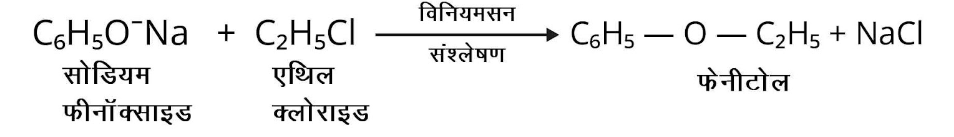(vi) $C{H_3}C{H_2}C{H_2}OH + SOC{l_2} \to$

उत्तर:

(vii) $C{H_3}C{H_2}CH = C{H_2} + HBr$ परॉक्साइड

उत्तर: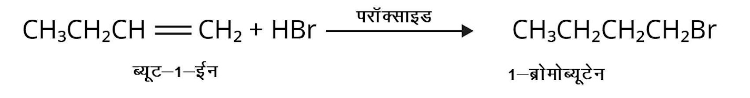(viii) $C{H_3}CH = C{\left( {C{H_3}} \right)_2} + HBr \to$

उत्तर: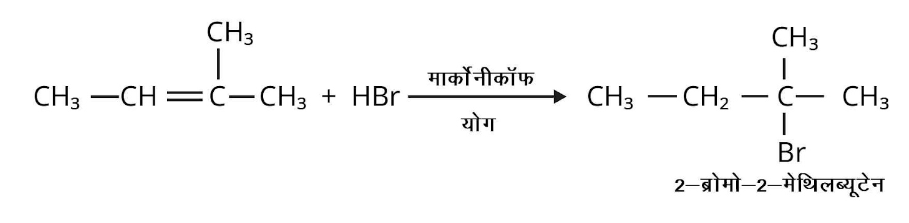15. निम्नलिखित अभिक्रिया की क्रियाविधि लिखिए – nBuBr + KCN $\underrightarrow { { EtOH-H }_{ 2 }O }$ nBuCN

उत्तर: KCN निम्न संरचनाओं का अनुनादी संकर होता है –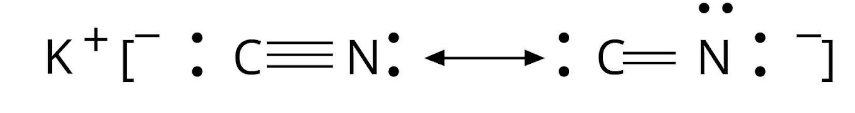अत: ${\text{CN--}}$उभयदन्ती नाभिकस्नेही के रूप में कार्य करता है। अतः यह ${\text{n -- BuBr}}$में ${\text{C - Br}}$आबन्ध के कार्बन परमाणु पर ${\text{C}}$या ${\text{N}}$परमाणु से आक्रमण करता है। चूंकि ${\text{C - N}}$आबन्ध, ${\text{C - C}}$आबन्ध से दुर्बल होता है अत: ${\text{C}}$पर आक्रमण होता है तथा ${\text{n - }}$ब्यूटिल सायनाइड बनता है।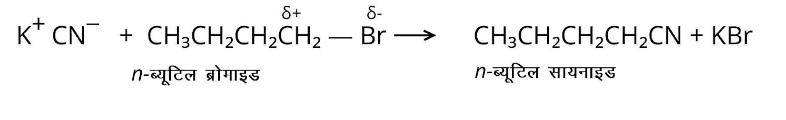16. ${\text{SN 2}}$प्रतिस्थापन के प्रति अभिक्रियाशीलता के आधार पर इन यौगिकों के समूहों को क्रमबद्ध कीजिए –

1. $2 -$ब्रोमो$- 2 -$मेथिलब्यूटेन, $1 -$ब्रोमोपेन्टेन, $2 -$ब्रोमोपेन्टेन

उत्तर: $1 -$ब्रोमोपेन्टेन > $2 -$ब्रोमोपेन्टेन > $2 -$ब्रोमो$- 2 -$मेथिलब्यूटेन

1. $1 -$ब्रोमो$- 3 -$मेथिलब्यूटेन, $2 -$ब्रोमो$- 2 -$मेथिलब्यूटेन, $2 -$ब्रोमो$- 3 -$मेथिलब्यूटेन

उत्तर: $1 -$ब्रोमो-3-मेथिलब्यूटेन > $2 -$ब्रोमो-3-मेथिलब्यूटेन > $2 -$ब्रोमो$- 2 -$मेथिलब्यूटेन

1. $1 -$ब्रोमोब्यूटेन, $1 -$ब्रोमो$- 2$, $2 -$डाइमेथिलप्रोपेन, $1 -$ब्रोमो$- 2 -$मेथिलब्यूटेन, $1 -$ब्रोमो$- 3 -$मेथिलब्यूटेन।

उत्तर: $1 -$ब्रोमोब्यूटेन > $1 -$ब्रोमो$- 3 -$मेथिलब्यूटेन > $1 -$ब्रोमो$- 2 -$ मेथिलब्यूटेन, $1 -$ब्रोमो$- 2$, $2 -$डाइमेथिलप्रोपेन

17. ${C_6}{H_5}C{H_2}Cl$ तथा $C{}_6{H_5}CHCl{C_6}{H_5}$में से कौन-सा यौगिक जलीय ${\text{KOH}}$ से , शीघ्रता से जल-अपघटित होगा?

उत्तर:

• SN 1 परिस्थितियों में $C{}_6{H_5}CHCl{C_6}{H_5}$,${C_6}{H_5}C{H_2}Cl$ की तुलना में शीघ्रता से जल अपघटित होगा।

• SN 2 परिस्थितियों में ${C_6}{H_5}C{H_2}Cl$,$C{}_6{H_5}CHCl{C_6}{H_5}$ की तुलना में शीघ्रता से जल-अपघटित होगा।

18. $o -$ तथा $m -$ समावयवियों की तुलना में $p -$ डाइक्लोरोबेन्जीन का गलनांक उच्च एवं विलेयता निम्न होती है, विवेचना कीजिए।

उत्तर: $p -$समावयव अधिक सममिताकार होने के कारण क्रिस्टल जालक में भली-भाँति स्थित हो जाता है, इसलिए इसमें $o -$ तथा $m -$ समावयवों की तुलना में प्रबल अन्तराअणुक आकर्षण बल उपस्थित होते हैं। चूँकि संगलन अथवा विलायकीकरण (solvation) के दौरान क्रिस्टल जालक टूटता है, इसलिए $p -$ समावयव के संगलन अथवा इसे विलेय करने के लिए सम्बन्धित $o -$ तथा $m -$ समावयवों की तुलना में अधिक ऊष्मा की आवश्यकता होती है। दूसरे शब्दों में, $p -$ समावयव का गलनांक सम्बन्धित $o -$ तथा $m -$ समावयव की तुलना में उच्च होता है, जबकि इसकी विलेयता निम्न होती है।

19. निम्नलिखित परिवर्तन कैसे सम्पन्न किए जा सकते हैं?

(i) प्रोपीन से प्रोपेन$- 1 -$ऑल

उत्तर: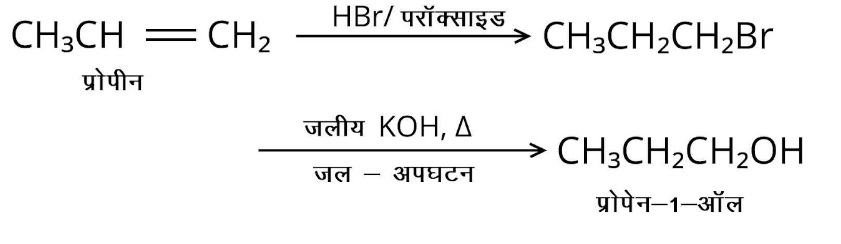(ii) एथेनॉल से ब्यूट$- 2 -$आइन

उत्तर: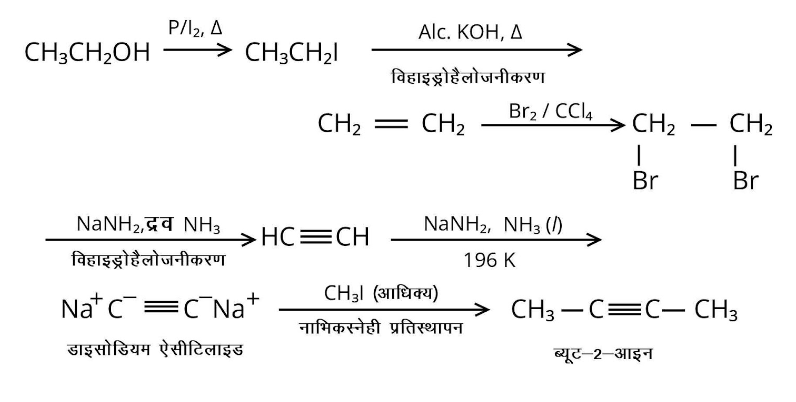(iii) $1 -$ब्रोमोप्रोपेन से $2 -$ब्रोमोप्रोपेन

उत्तर: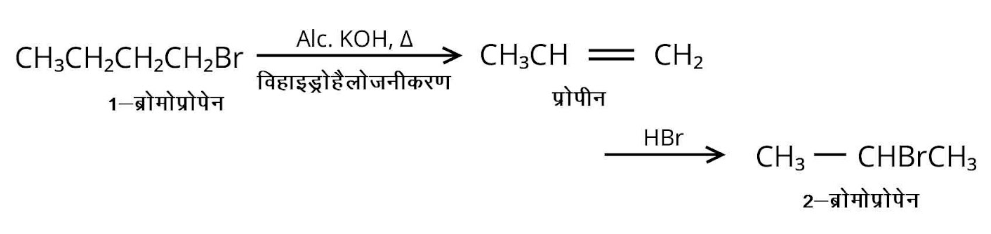(iv) टॉलूईन से बेन्जिल ऐल्कोहॉल।

उत्तर: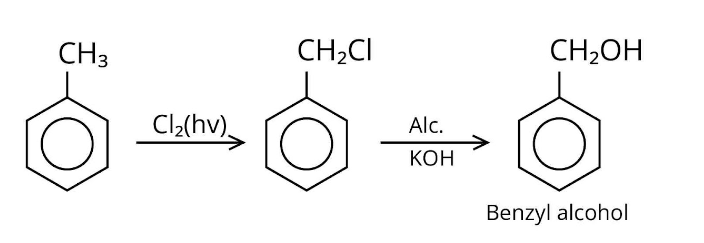(v) बेन्जीन से $4 -$ ब्रोमोनाइट्रोबेन्जीन

उत्तर: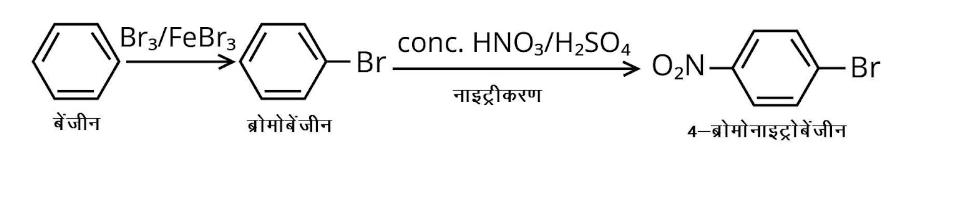(vi) बेन्जिल ऐल्कोहॉल से $2 -$फेनिल एथेनोइक अम्ल

उत्तर: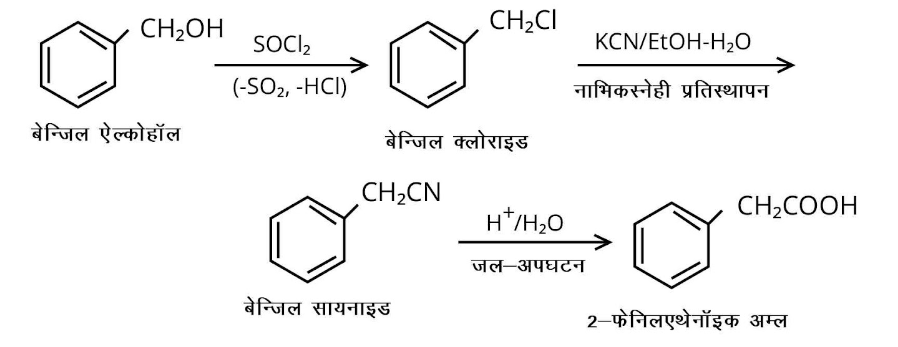(vii) एथेनॉल से प्रोपेननाइट्राइल

उत्तर: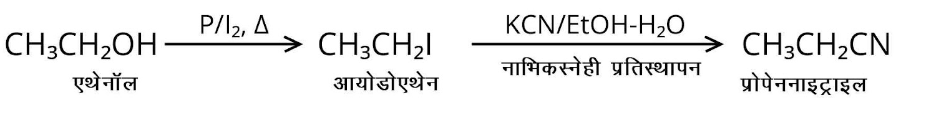(viii) ऐनिलीन से क्लोरोबेन्जीन

उत्तर: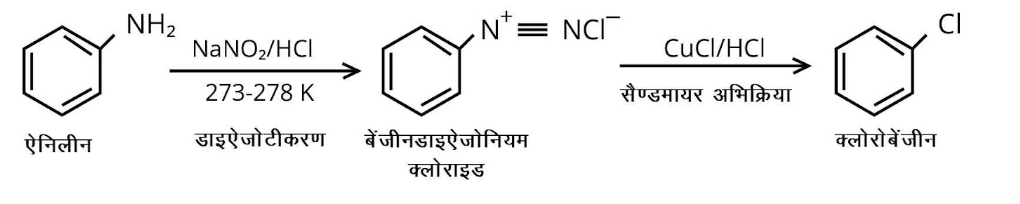(ix) $2 -$क्लोरोब्यूटेन से $3,4 -$डाइमेथिलहेक्सेन

उत्तर: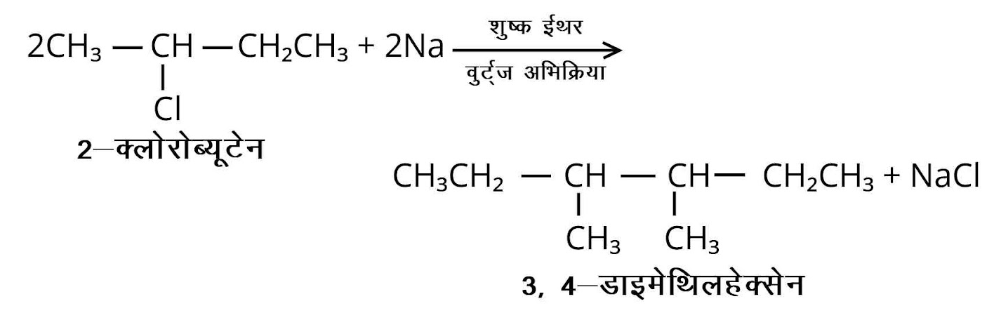(x) $2 -$मेथिल$- 1 -$प्रोपीन से $2 -$क्लोरो$- 1 -$मेथिलप्रोपेन

उत्तर: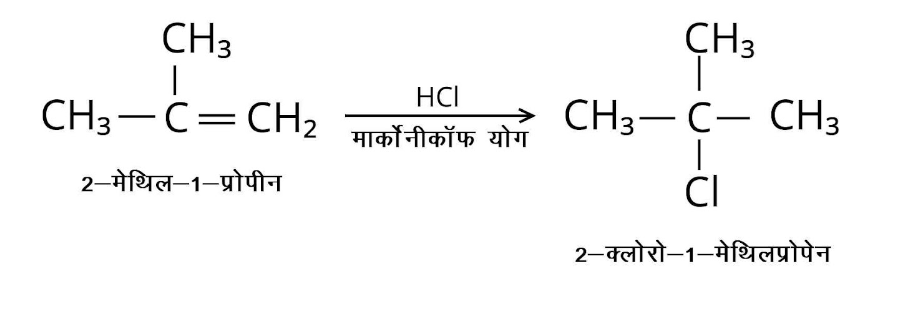(xi) एथिल क्लोराइड से प्रोपेनोइक अम्ल

उत्तर:

(image will be updated soon)

(xii) ब्यूट$- 1 -$ईन से ${\text{n - }}$ब्यूटिल आयोडाइड

उत्तर: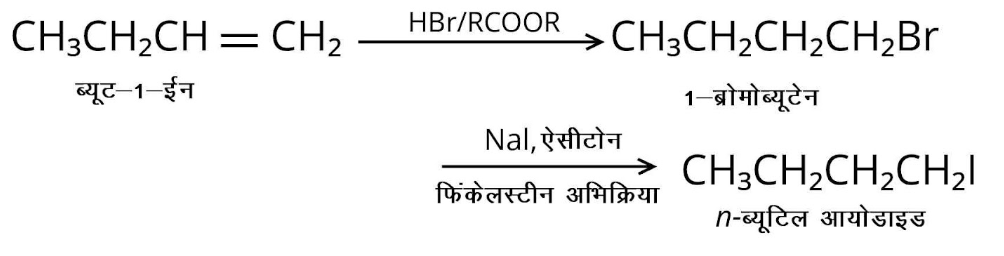(xiii) $2 -$क्लोरोप्रोपेन से $1 -$प्रोपेनॉल

उत्तर: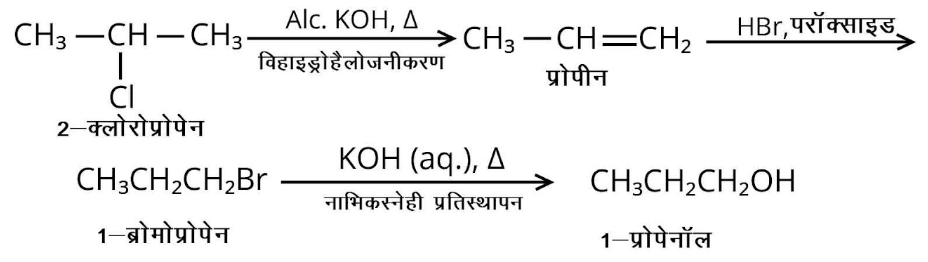(xiv) आइसोप्रोपिल ऐल्कोहॉल से आयोडोफॉर्म

उत्तर: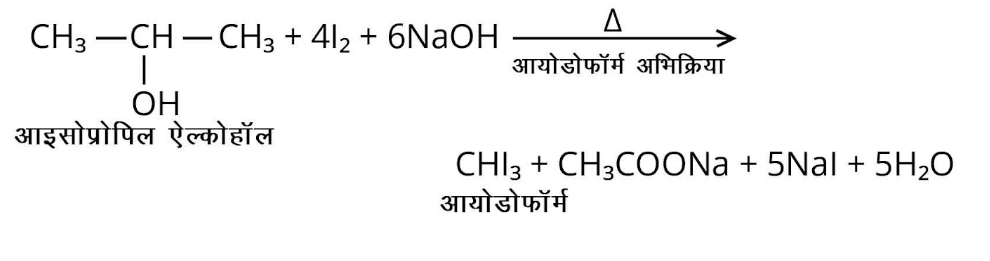(xv) क्लोरोबेन्जीन से ${\text{p - }}$नाइट्रोफीनॉल

उत्तर:

(xvi) $2 -$ब्रोमोप्रोपेन से ${\mathbf{1}} -$ब्रोमोप्रोपेन

उत्तर: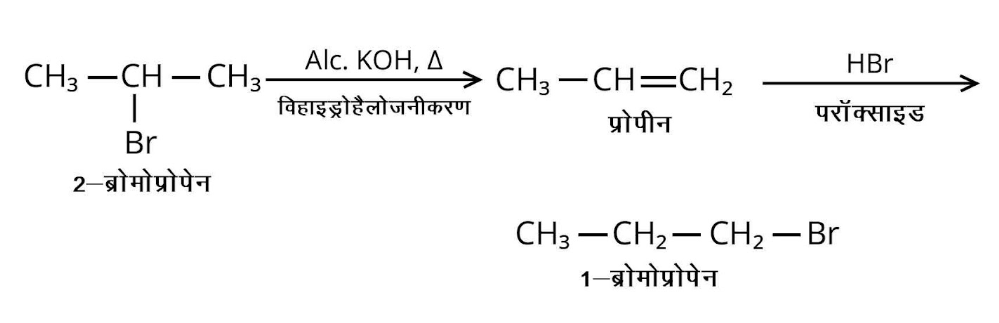(xvii) क्लोरोएथेन से ब्यूटेन

उत्तर: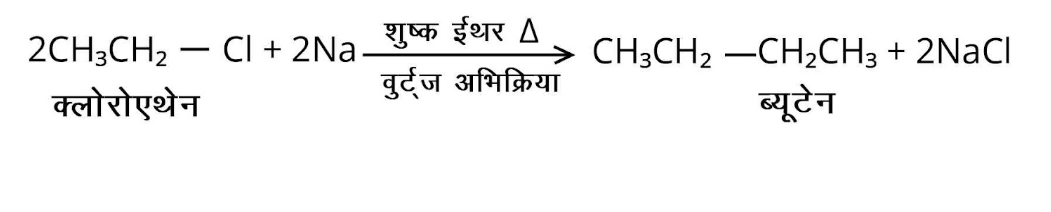(xviii) बेन्जीन से डाइफेनिल

उत्तर:(xix) तृतीयक-ब्यूटिल ब्रोमाइड से आइसो-ब्यूटिल ब्रोमाइड

उत्तर: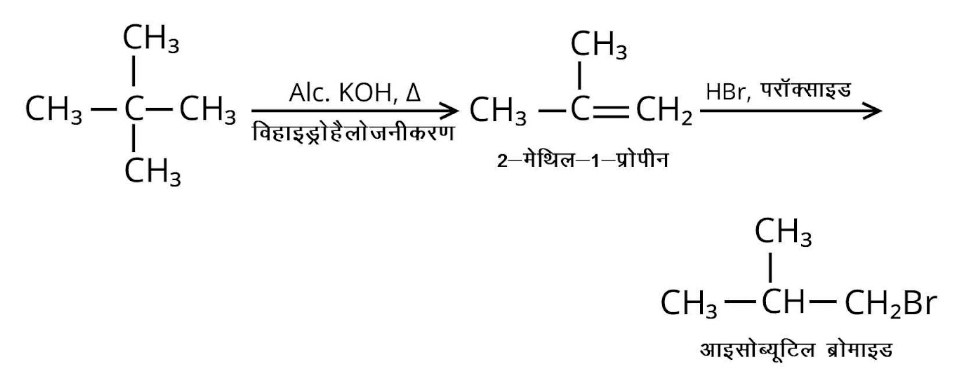(xx) ऐनिलीन से फेनिलआइसोसायनाइडे।

उत्तर: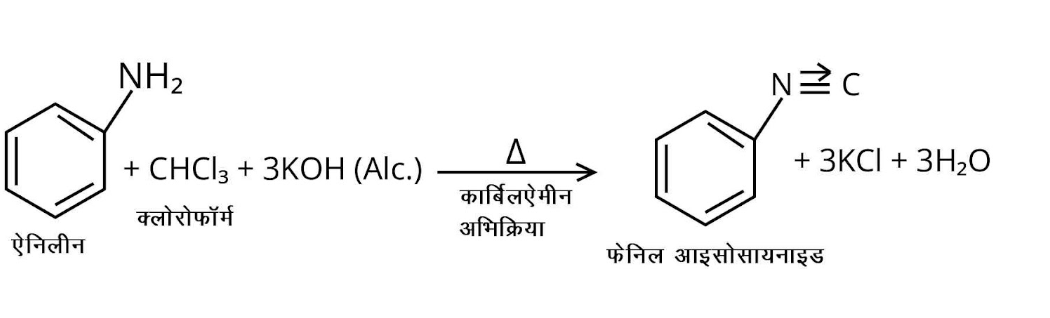20. ऐल्किल क्लोराइड की जलीय ${\text{KOH}}$ से अभिक्रिया द्वारा ऐल्कोहॉल बनता है, लेकिन ऐल्कोहॉलिक ${\text{KOH}}$ की उपस्थिति में ऐल्कीन मुख्य उत्पाद के रूप में प्राप्त होती है। समझाइए।

उत्तर: जलीय विलयन में ${\text{KOH}}$लगभग पूर्ण आयनित होकर ${\text{OH--}}$ आयन देता है जो प्रबल नाभिकरागी होने के कारण ऐल्किल हैलाइडों पर प्रतिस्थापन अभिक्रिया करके ऐल्कोहॉल बनाते हैं। जलीय विलयन में ${\text{OH--}}$ आयन उच्च जलयोजित होते हैं। इससे ${\text{OH--}}$ आयनों का क्षारीय गुण घट जाता है जिससे ये ऐल्किल हैलाइड के $\beta -$ कार्बन से हाइड्रोजन परमाणु पृथक्कृत करने में असफल हो जाते हैं तथा ऐल्कीन नहीं बना पाते। दूसरी ओर ${\text{KOH}}$ के ऐल्कोहॉली विलयन में ऐल्कॉक्साइड (${\text{RO--}}$) आयन होते हैं जो ${\text{OH--}}$से प्रबल क्षार होने के कारण सरलतापूर्वक ऐल्किल क्लोराइड से ${\text{HCl}}$अणु का विलोपन करके ऐल्कीन बना लेते हैं।

21. प्राथमिक ऐल्किल हैलाइड ${C_4}{H_9}Br$

(क), ऐल्कोहॉलिक ${\text{KOH}}$से अभिक्रिया द्वारा यौगिक

उत्तर: आण्विक सूत्र ${C_4}{H_9}Br$के दो प्राथमिक हैलाइड निम्नलिखित हो सकते हैं –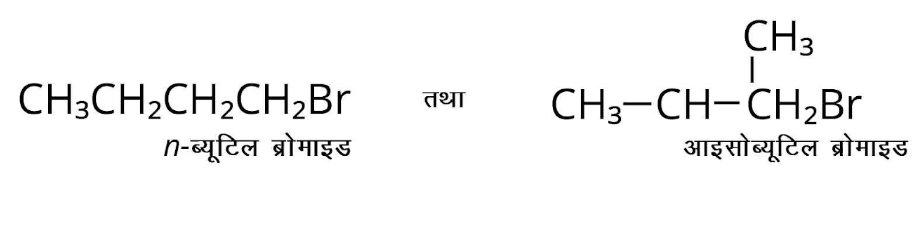अतः यौगिक

(क) या तो ${\text{n - }}$ ब्यूटिल ब्रोमाइड है या आइसोब्यूटिल ब्रोमाइड। चूँकि यौगिक ‘क’ की अभिक्रिया सोडियम धातु से होने पर यौगिक ‘घ’ (आण्विक सूत्र ${C_8}{H_{18}}$) प्राप्त होता है जो कि ${\text{n - }}$ब्यूटिल ब्रोमाइड की अभिक्रिया सोडियम धातु से होने पर प्राप्त यौगिक से भिन्न है, इसलिए यौगिक ‘क’ आइसोब्यूटिल ब्रोमाइड होना चाहिए तथा यौगिक ‘घ’ $2,5 -$डाइमेथिलहेक्सेन होना चाहिए।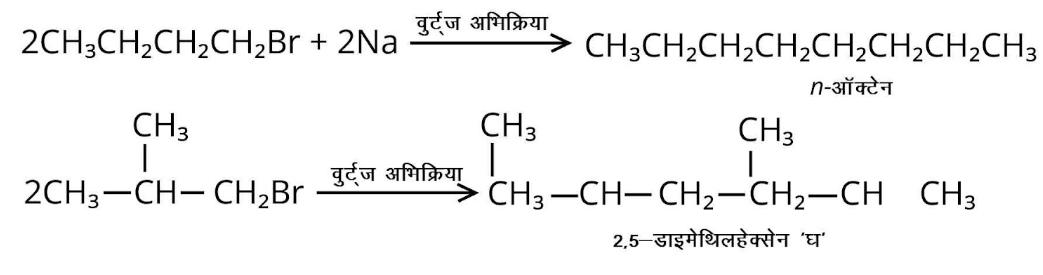अब यदि यौगिक ‘क’ आइसोब्यूटिल ब्रोमाइड है तो यौगिक ‘ख’, जो यौगिक ‘क’ की ऐल्कोहॉलिक ${\text{KOH}}$ से अभिक्रिया द्वारा प्राप्त होता है, $2 -$मेथिल$- 1 -$प्रोपीन होना चाहिए।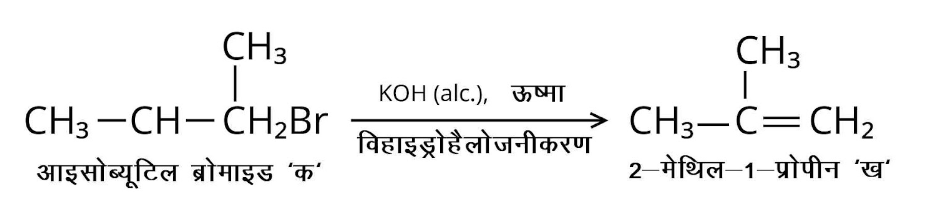(ख) यौगिक ‘ख’ ${\text{HBr}}$के साथ अभिक्रिया से यौगिक ‘ग देता है जो कि यौगिक ‘क’ का समावयवी है। जब यौगिक ‘क’ की अभिक्रिया सोडियम धातु से होती है तो यौगिक ‘घ’ $C8H18$बनता है, जो कि ब्यूटिल ब्रोमाइड की सोडियम से अभिक्रिया द्वारा बने उत्पाद से भिन्न है। यौगिक ‘क’ का संरचना सूत्र दीजिए तथा सभी अभिक्रियाओं की समीकरण दीजिए।

उत्तर: यौगिक ‘ख’ HBr के साथ अभिक्रिया से मार्कोनीकॉफ नियम के अनुसार यौगिक ‘ग’ देता है। इसलिए यौगिक ‘ग’ तृतीयक-ब्यूटिल ब्रोमाइड है जो यौगिक ‘क’ (आइसोब्यूटिल ब्रोमाइड) का एक समावयव है।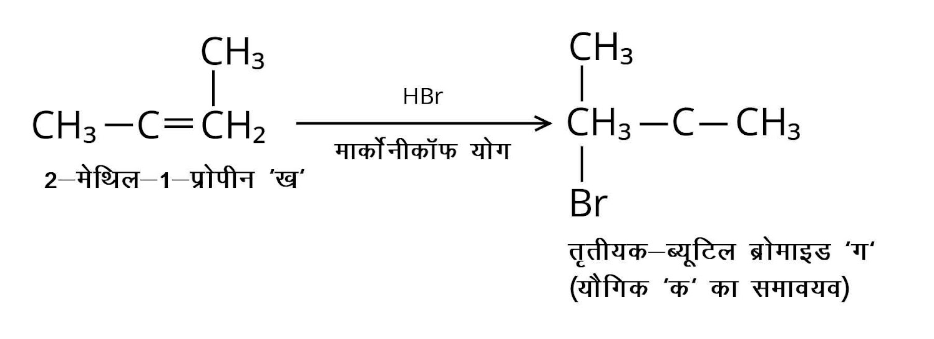इस प्रकार, ‘क’ आईसोब्यूटिल ब्रोमाइड, ‘ख’ $2 -$मेथिल$- 1 -$प्रोपीन, ‘ग’ तृतीयक-ब्यूटिल ब्रोमाइड तथा ‘घ’ $2,5 -$डाइमेथिलहेक्सेन है।

22. तब क्या होता है जब –

(i) ${\text{n - }}$ ब्यूटिल क्लोराइड को ऐल्कोहॉलिक ${\text{KOH}}$ के साथ अभिकृत किया जाता है?

उत्तर: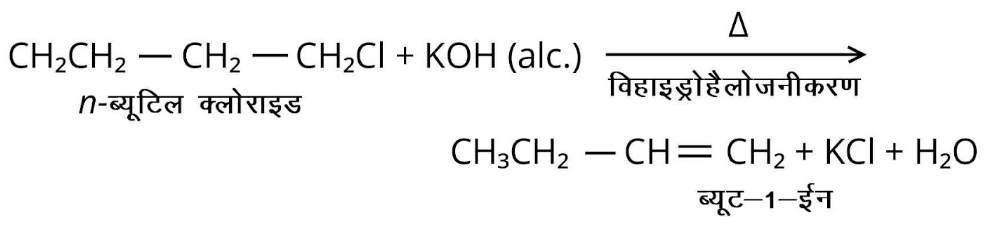(ii) शुष्क ईथर की उपस्थिति में ब्रोमोबेन्जीन की अभिक्रिया मैग्नीशियम से होती है?

उत्तर: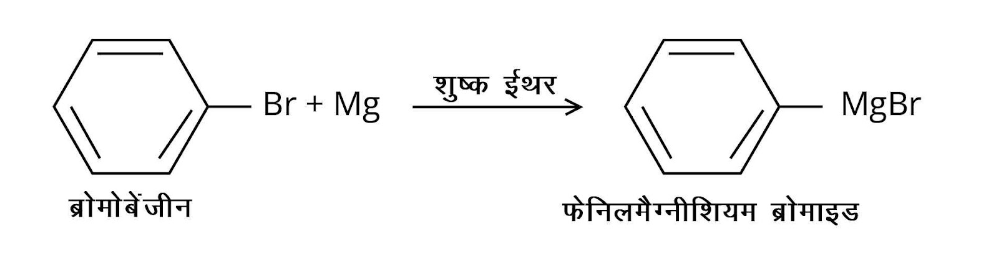(iii) क्लोरोबेन्जीन का जल-अपघटन किया जाता है?

उत्तर: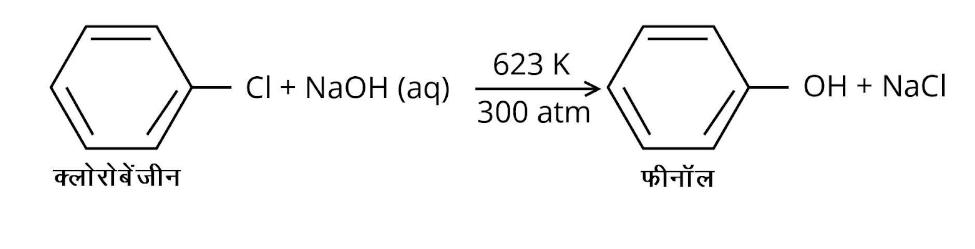(iv) एथिल क्लोराइड की अभिक्रिया जलीय ${\text{KOH}}$ से होती है?

उत्तर: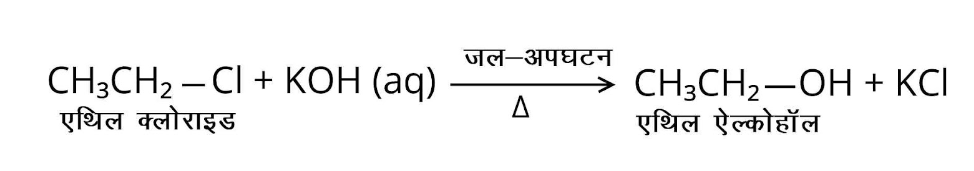(v) शुष्क ईथर की उपस्थिति में मेथिल ब्रोमाइड की अभिक्रिया सोडियम से होती है?

उत्तर: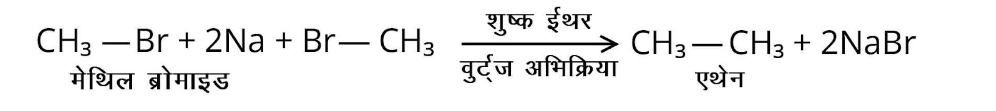(vi) मेथिल क्लोराइड की अभिक्रिया ${\text{KCN}}$से होती है?

उत्तर: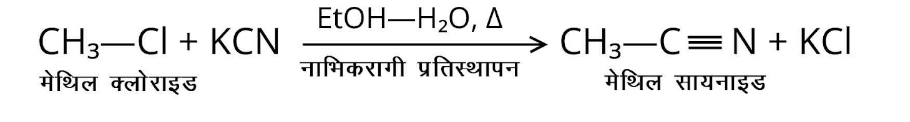### NCERT Solutions for Class 12 Chemistry Chapter 10 Haloalkanes and Haloarenes in Hindi Mediem

Chapter-wise NCERT Solutions are provided everywhere on the internet with an aim to help the students to gain a comprehensive understanding. Class 12 Chemistry Chapter 10 solution Hindi medium are created by our in-house experts keeping the understanding ability of all types of candidates in mind. NCERT textbooks and solutions are built to give a strong foundation to every concept. These NCERT Solutions for Class 12 Chemistry Chapter 10 in Hindi ensure a smooth understanding of all the concepts including the advanced concepts covered in the textbook.

NCERT Solutions in Hindi medium have been created keeping those students in mind who are studying in a Hindi medium school. These NCERT Solutions for Class 12 Chemistry Haloalkanes and Haloarenes in Hindi medium pdf download have innumerable benefits as these are created in simple and easy-to-understand language. The best feature of these solutions is a free download option. Students of Class 12 can download these solutions at any time as per their convenience for self-study purpose.

These solutions are nothing but a compilation of all the answers to the questions of the textbook exercises. The answers/ solutions are given in a stepwise format and very well researched by the subject matter experts who have relevant experience in this field. Relevant diagrams, graphs, illustrations are provided along with the answers wherever required. In nutshell, NCERT Solutions for Class 12 Chemistry in Hindi come really handy in exam preparation and quick revision as well prior to the final examinations.

## FAQs on NCERT Solutions for Class 12 Chemistry Chapter 10 - In Hindi

1. List out the sub-topics covered in Chapter 10 of NCERT Solutions for Class 12 Chemistry?

Class 12 Chemistry Chapter 10 talks about Haloalkanes and Haloarenes. Chapter 10 of NCERT Solutions for Class 12 Chemistry includes all the sub-topics available in the NCERT books. Some of these sub-topics include Classification, Nomenclature, Nature of C-X Bond, Methods of Preparation, Physical Properties, Chemical Reactions, and Polyhalogen Compounds. The materials for these sub-topics are provided by Vedantu. The topics are explained in an easy language making it easier for the students to understand the syllabus.

2. What are the important topics of this chapter?

Chapter 10 of CBSE Class 12 Chemistry has several important topics from the examination point of view.  There are topics such as Classification, Nomenclature, Nature of C-X Bond, Methods of Preparation, Physical Properties, Chemical Reactions, Optical Rotation, Uses and environmental effects of; Dichloromethane, Trichloromethane, Tetrachloromethane, Iodoform, Freons, DDT, and Polyhalogen Compounds. Students will become efficient in such concepts only through revision. The more questions they solve, the better they will become at the subject.

3. What is the weightage of NCERT class 12 Chemistry Chapter 10 in the CBSE board exam?

From the board examination point of view, students need to look at NCERT Solutions to understand the chapter better. Class 12 Chemistry Chapter 10 shares the marks weightage of Chapters 11, 12, 13, and 14. Around 28 marks worth of questions are asked from these chapters. However, this may vary every year. There are practice question papers and exercises for students to use to prepare and understand the board question paper pattern better.

4. How do the NCERT Solutions for Class 12 Chemistry Chapter 10 PDF help students?

All the students rely on NCERT Solutions to ace the subject in their board examinations. With the help of NCERT Solutions for Class 12 Chemistry Chapter 10 PDF, students can learn all the concepts available in the chapter easily with practice questions and exercises available. These PDFs are available at free of cost on the official website of Vedantu and on the Vedantu app. There are practice question papers and exercises for students to use to prepare and understand the chapter better. The more questions they solve, the better they will become at the subject.

5. What are the basics of Haloalkanes and Haloarenes?

Haloalkanes and Haloarenes are hydrocarbon compounds that consist of one or more hydrogen atoms replaced by Halogen atoms. These compounds can contain wide ranges of Halogen atoms. The main difference between Haloalkanes and Haloarenes is that Haloalkanes come from nothing but open-chain hydrocarbons, which are also known as Alkanes. While Haloarenes comes from aromatic hydrocarbons, Haloalkanes are generally referred to as alkyl, and Haloarenes are commonly known as aryl halides.